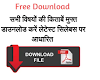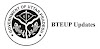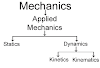UP polytechnic 2nd Semester Applied Physics 2 Notes | Engineering Physics PDF

What You Learn In Applied Physics 2 Notes Engineering Physics PDF?

Define wave motion its types (Transverse and Longitudinal), Periodic and Simple Harmonic Motion, solve simple problems.

Define the terms: frequency, amplitude, wavelength, velocity of a wave.

Explain various Engineering Physics PDF, Medical and Industrial applications of Ultrasonic’s.

Apply acoustics principles to various types of buildings to get best sound effect

Explain diffraction, interference, polarization.

Define capacitance and its unit. They will be able to explain the function of capacitors in simple circuits, solve simple problems using C=Q/V

Explain the role of free electrons in insulators, conductors and semiconductors, qualitatively the terms: potential, potential difference, electromotive force.

Explain the concept of electric current, resistance and its measurement.

List the effects of an electric current and their common applications, State and apply Ohm’s law, calculate the equivalent resistance of a variety of resistor combinations, determine the energy consumed by an appliance, differentiate between AC electricity and DC electricity

Explain Biot-Savart Law, Ampere’s law, Lorenz Force.

State the laws of electromagnetic induction, describe the effect on a current-carrying conductor when placed in a magnetic field Explain operation of moving coil galvanometer, simple DC motor

Apply the knowledge of diodes in rectifiers, adapters IC’s and various electronic circuits. Apply the concept of light amplification in designing of various LASER based instruments and optical sources.

Explain total internal reflection and apply this concept for optical fibre and its uses in Medical field and Communication.

List Of Chapter And Details Topic In Applied Physics 2 Notes Engineering Physics PDF Up Polytechnic

Unit 1. Wave motion and its applications

1.1 Wave motion, transverse and longitudinal wave motion with examples, sound and light waves, relationship among wave velocity, frequency and wave length and its application

1.2 Wave equation y = r sin wt, phase, phase difference, principle of superposition of waves.

List Of Practical in Applied Physics 2 Notes Up Polytechnic | Engineering Physics PDF | Up Polytechnic (Perform Minimum Six Experiments)

• To determine the velocity of sound with the help of resonance tube.
• Demonstrate To find focal length of the convex lens by the displacement method.
• To find the refractive index of the material of given prism using spectrometer.
• To find the wavelength of sodium light using Fresnel’s biprism.
• To verify laws of resistances in series and parallel combination
• To verify ohm’s laws by drawing a graph between voltage and current.
• To measure very low resistance and very high resistances using Slide Wire bridge

## Books Recommended For Applied Physics 2 Notes Engineering Physics PDF In Up Polytechnic 2nd Semester.

• Text Book of Physics (Part-I, Part-II); N.C.E.R.T., Delhi
• Concepts in Physics by HC Verma, Vol. I & II, Bharti Bhawan Ltd. New Delhi
• A Text Book of Optics, written by Subramanian and Brij Lal, S Chand & Co., New Delhi
• Practical Physics, by C. L. Arora, S Chand publications
• Engineering Physics PDF Physics by PV Naik, Pearson Education Pvt. Ltd, New Delhi
• Modern Engineering Physics PDF Physics by SL Gupta, Sanjeev Gupta, Dhanpat Rai Publications.
• Physics Volume 2, 5th edition, Haliday Resnick and Krane, Wiley publication
• Fundamentals of Physics by Haliday, Resnick & Walker 7th edition, Wiley publication
• Up Polytechnic 2nd Semester Applied Physics – 2 Notes in Hindi

Previous article
Next article

### Trending Posts